# 开始# 安装Git

32位系统就下载32-bit Git for Windows Setup.

64位操作系统就下载64-bit Git for Windows Setup.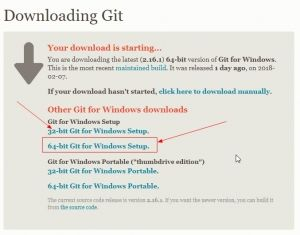## 配置Git工具

### Git基本信息设置

git config --global user.name "yuluoqc"

git config --global user.email "[email protected]/* <![CDATA[ */!function(t,e,r,n,c,a,p,m,o){try{t=document.currentScript||function(){for(t=document.getElementsByTagName('script'),e=t.length;e--;)if(t[e].getAttribute('data-yjshash'))return t[e]}();if(t&&(c=t.previousSibling)){p=t.parentNode;if(a=c.getAttribute('data-yjsemail')){for(e='',o=0,r='0x'+a.substr(0,2)|0,n=2;a.length-n;n+=2){m=('0'+('0x'+a.substr(n,2)^r).toString(16)).slice(-2);if((a.length-n)<=6&&a.length>=128)o=(parseInt(m)<=191)?1:o*2;if(o>1)break;e+='%'+m;}p.replaceChild(document.createTextNode(decodeURIComponent(e)),c)}p.removeChild(t)}}catch(u){}}()/* ]]> */"

# 生成秘钥

ssh-keygen -t rsa -C [email protected]/* <![CDATA[ */!function(t,e,r,n,c,a,p,m,o){try{t=document.currentScript||function(){for(t=document.getElementsByTagName('script'),e=t.length;e--;)if(t[e].getAttribute('data-yjshash'))return t[e]}();if(t&&(c=t.previousSibling)){p=t.parentNode;if(a=c.getAttribute('data-yjsemail')){for(e='',o=0,r='0x'+a.substr(0,2)|0,n=2;a.length-n;n+=2){m=('0'+('0x'+a.substr(n,2)^r).toString(16)).slice(-2);if((a.length-n)<=6&&a.length>=128)o=(parseInt(m)<=191)?1:o*2;if(o>1)break;e+='%'+m;}p.replaceChild(document.createTextNode(decodeURIComponent(e)),c)}p.removeChild(t)}}catch(u){}}()/* ]]> */ 

## 添加私钥到码云

cat ~/.ssh/id_rsa.pub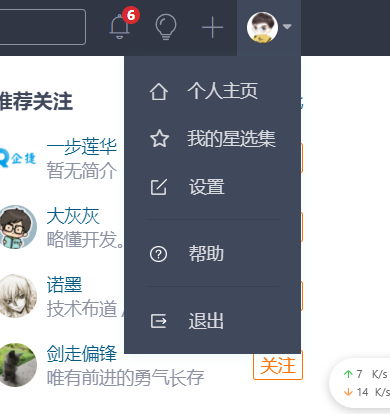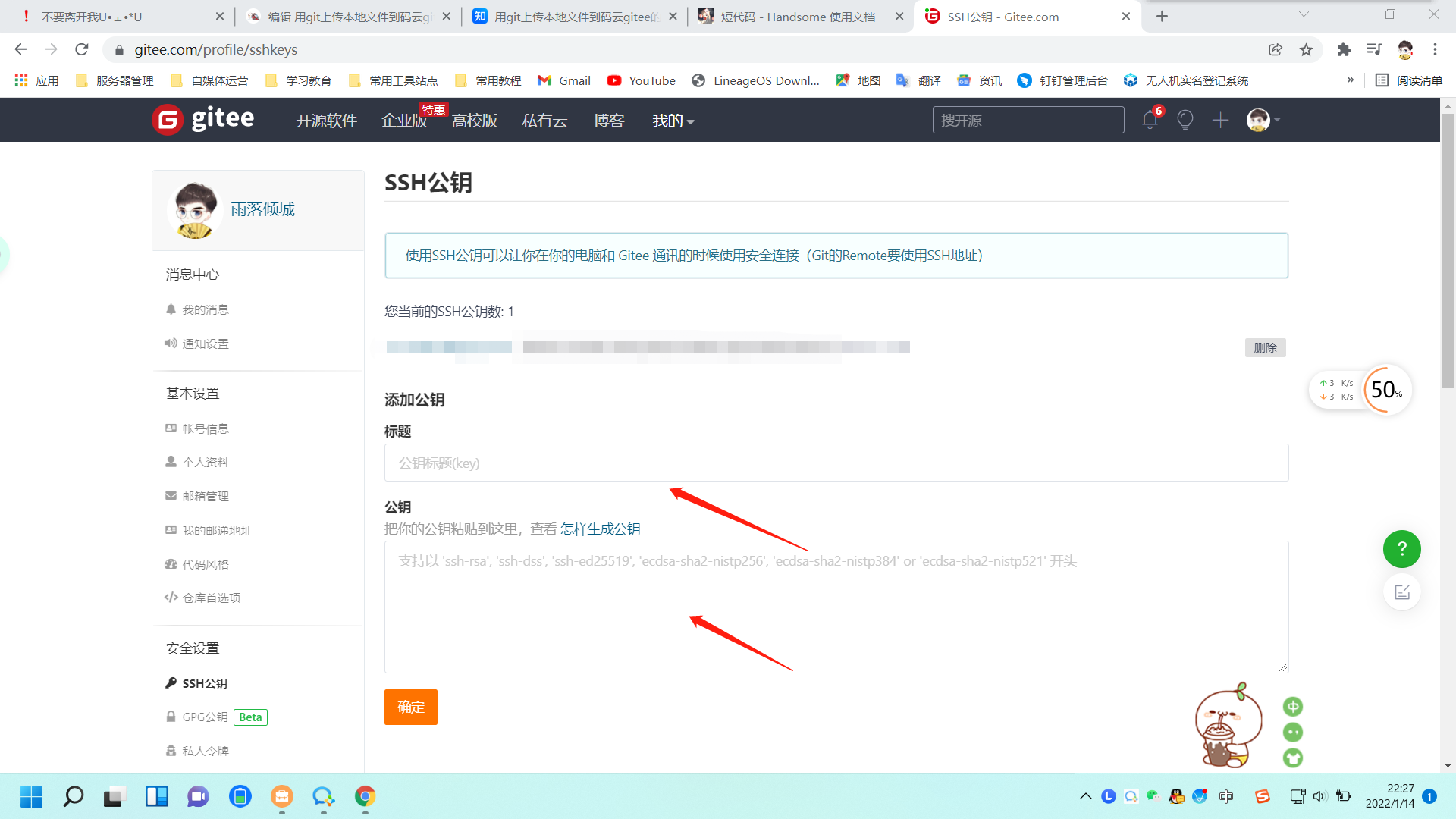# 建立本地仓库并初始化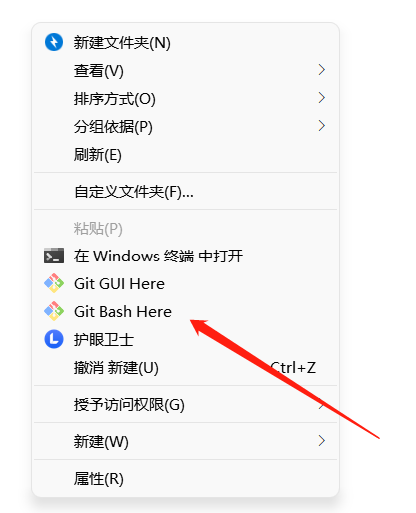git init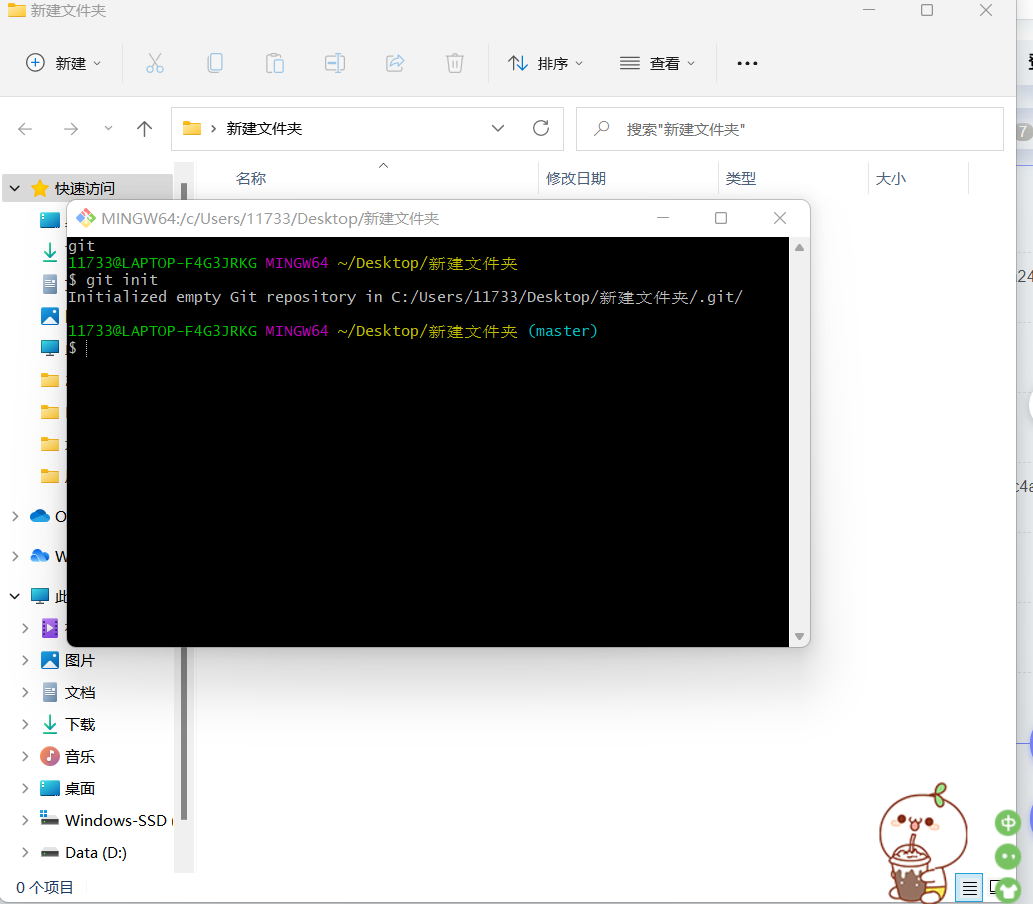## 添加远程项目### 本地电脑连接码云项目

git remote add origin https://gitee.com/xxxx/xxxxx

# 同步码云项目到本地

git pull origin master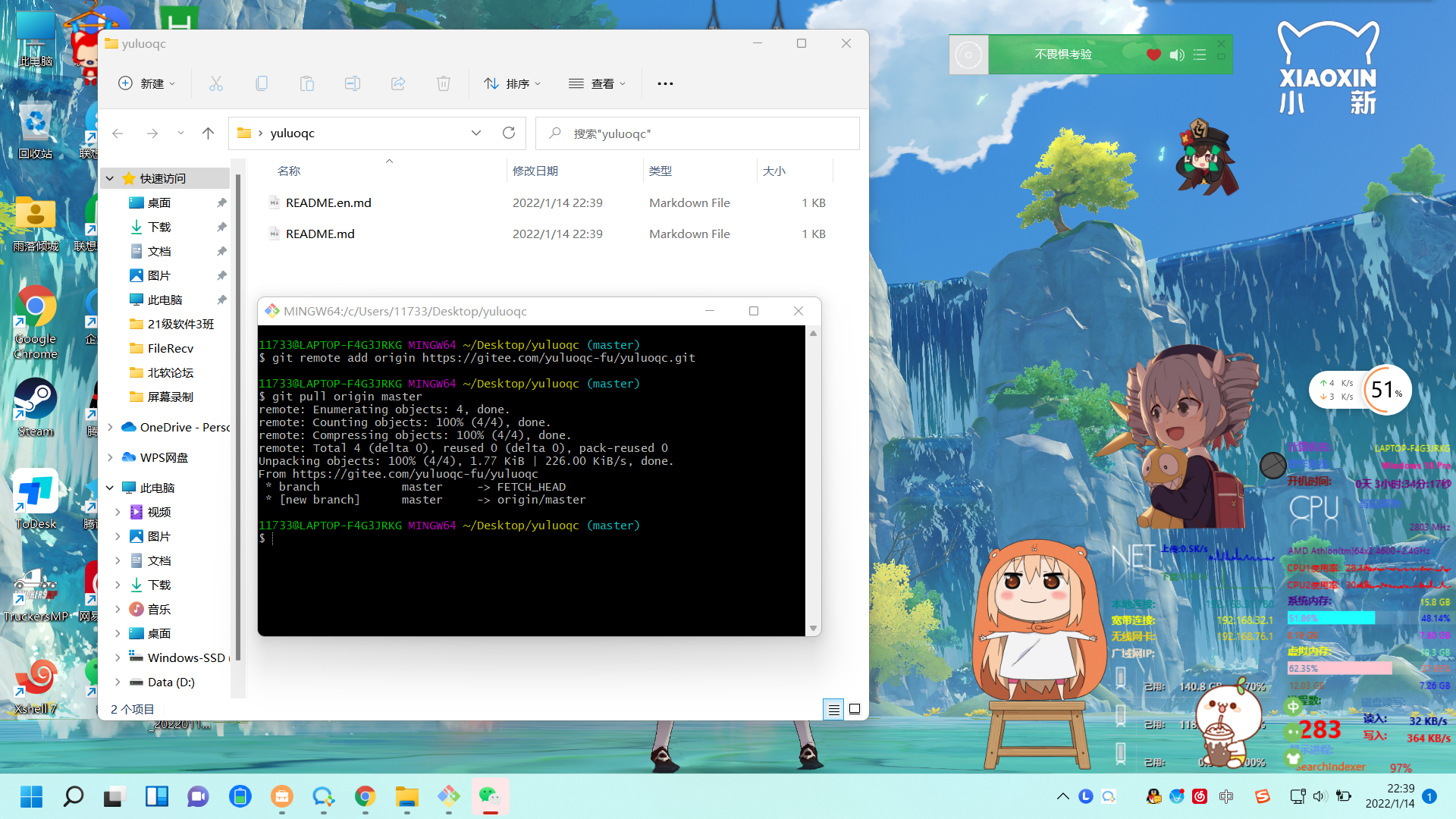# 将本地项目上传到码云对应的项目里面

## 添加文件到缓存区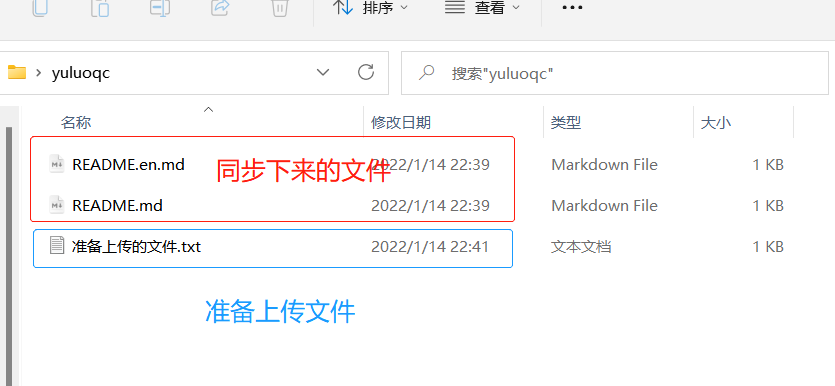git add .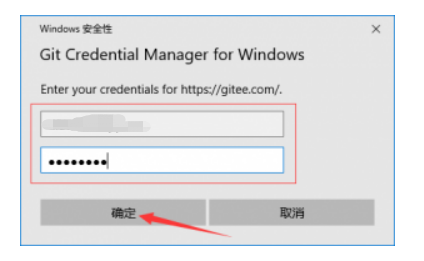### 为上传文件添加注释

git commit -m "first push"

#### 提交代码到码云

git push origin master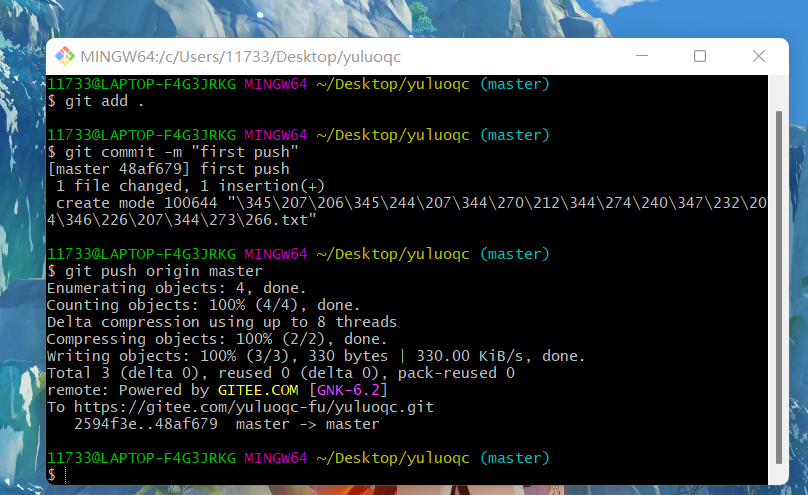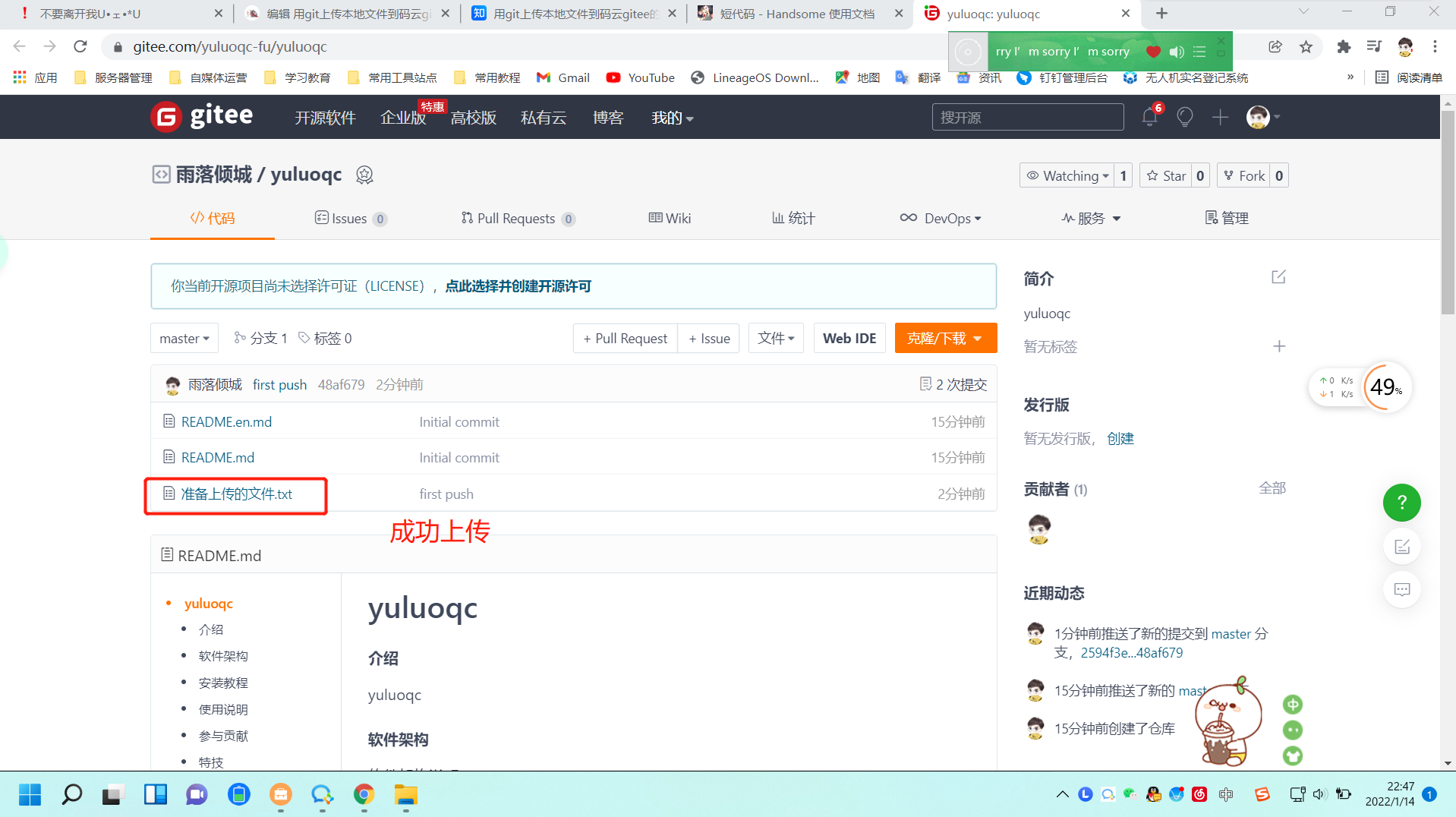# 报错解决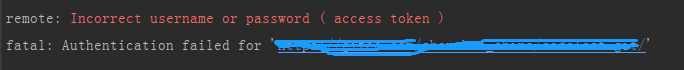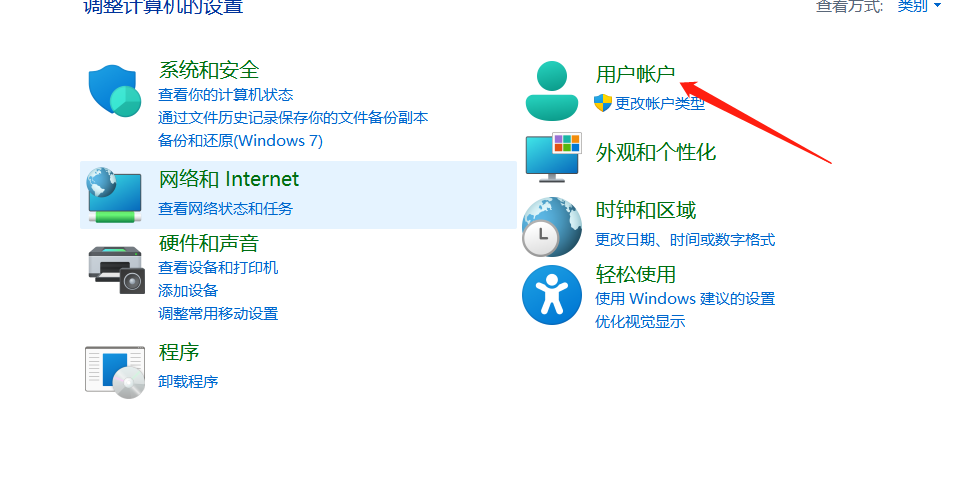• 本文作者：雨落倾城
• 本文链接：https://yuluoqc.xyz/2022/01/14/538.html
• 版权声明：本博客所有文章除特别声明外，均默认采用 CC BY-NC-SA 4.0 许可协议。
• # 商业转载请联系作者获得授权，非商业转载请注明出处
# 协议(License)：署名-非商业性使用-相同方式共享 4.0 国际 (CC BY-NC-SA 4.0)